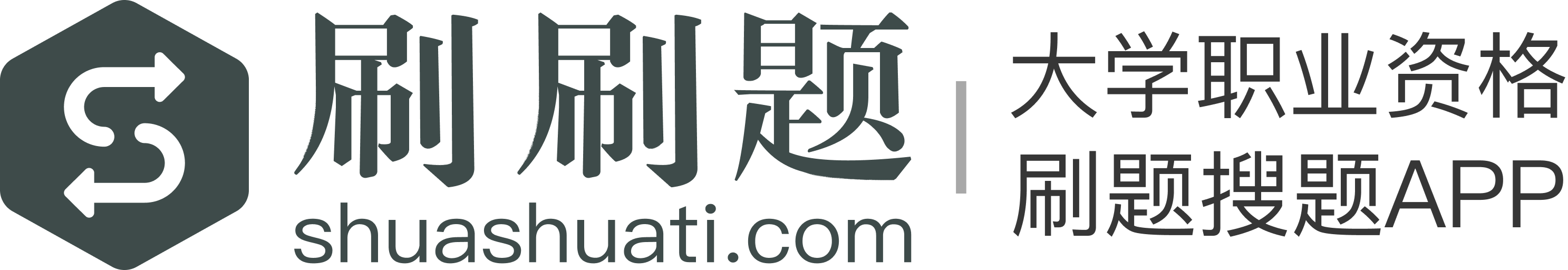【单选题】# 下面方程的图像是函数的是

A.
$x^2+y^2=1$
B.
$x^4+y^4=1$
C.
$\sin (xy)=\frac{1}{2}$
D.
$|x+1|+|y-1|=0$A.

B.

C.

D.

## 【单选题】下面关于钠泵生理意义的叙述，错误的是（）

A.

B.

C.

D.
Na + 在膜两侧的浓度差是其他许多物质继发性主动转运（如葡萄糖、氨基酸等）的动力
E.

## 【单选题】下面哪一项不是面试的特点？（ ）

A.

B.

C.

D.打开“刷刷题APP”, 刷题搜题更便捷